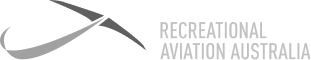# Definition: Angle of Bank

This is the angle of the lateral axis of the aircraft to the horizontal plane. In other words, it is the angle of the wings to the horizon when viewed from the rear. If wings are level with the horizon, AoB will be 0 degrees. If the wings are in a vertical position to the horizon, the AoB will be 90 degrees.### Angle of Bank

This is the angle of the lateral axis of the aircraft to the horizontal plane. In other words, it is the angle of the wings to the horizon when viewed from the rear. If wings are level with the horizon, AoB will be 0 degrees. If the wings are in a vertical position to the horizon, the AoB will be 90 degrees.

7 Day

FREE

Trial